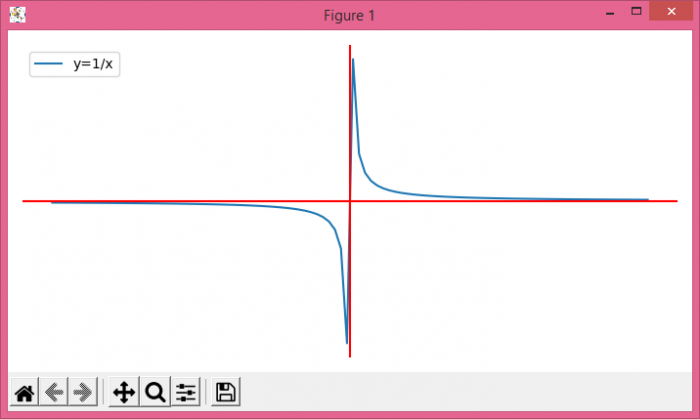# How to handle an asymptote/discontinuity with Matplotlib?

MatplotlibPythonData Visualization

#### Python Data Science basics with Numpy, Pandas and Matplotlib

Most Popular

63 Lectures 6 hours

#### Data Visualization using MatPlotLib & Seaborn

11 Lectures 4 hours

#### MatPlotLib with Python

9 Lectures 2.5 hours

To handle an asymptote/discontinuity with matplotlib, we can take the following steps −

• Create x and y data points using numpy.

• Turn off the axes plot.

• Plot the line with x and y data points.

• Add a horizontal line across the axis, x=0.

• Add a vertical line across the axis, y=0.

• Place legend for the curve y=1/x.

• To display the figure, use show() method.

## Example

import numpy as np
from matplotlib import pyplot as plt
plt.rcParams["figure.figsize"] = [7.00, 3.50]
plt.rcParams["figure.autolayout"] = True
x = np.linspace(-1, 1, 100)
y = 1 / x
plt.axis('off')
plt.plot(x, y, label='y=1/x')
plt.axhline(y=0, c='red')
plt.axvline(x=0, c='red')
plt.legend(loc='upper left')
plt.show()

## Output